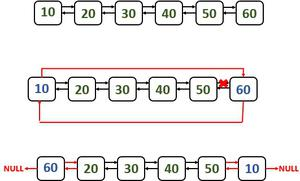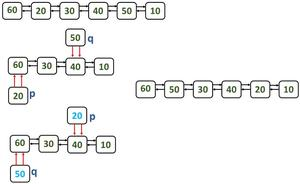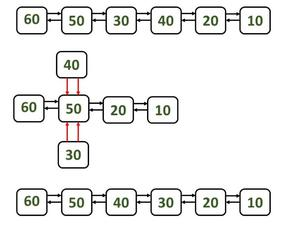Swap Kth node from beginning with Kth node from end in a Doubly Linked List

• Difficulty Level : Hard
• Last Updated : 15 Apr, 2021

Given a doubly-linked list, the task is to swap Kth node from the beginning with Kth node from the ending.
Note: Please note here the nodes are swapped and not the data in the nodes.
Examples:

Input: DLL = 1 <-> 2 <-> 3 <-> 4 <-> 5 <-> 6, K = 3
Output: 1 2 4 3 5 6
Explanation:
Third node from the beginning(3) is swapped with third node from the ending(4).
Input: DLL = 1 <-> 2 <-> 3 <-> 4 <-> 5, K = 1
Output: 5 2 3 4 1

Approach: The idea is to traverse to the Kth element from the beginning and Kth node from the ending and change the previous and next pointers. Let K1 be the Kth node from beginning and K2 be Kth node from ending. Then:

• The previous node to K2 has to be changed to the previous node of K1.
• The next node to K2 has to be changed to the next node of K1.
• The previous node to K1 has to be changed to the previous node of K2.
• The next node to K1 has to be changed to the next node of K2.

Below is the implementation of the above approach:

C#

Output:
1 4 3 2 5

Method 2: Without swapping the elements and without using temporary node.

Approach: There are 3 cases in order to swap the nodes.

• Swapping the first and the last nodes (k = 1)
• Swapping the ordinary Kth node from the beginning and Kth node from the end.
• Swapping middle nodes

Case 1: Swap first and last nodes (k = 1)

Steps:

1. Make the list as a circular linked list
2. Change the previous pointer of the first node to the last but one node (20 in example figure)
3. Change the next pointer of last but one node to the last node. In this case it will be 60.
4. After swapping, make the head as the first node.
Consider p and q are the nodes which are to be swapped,

last = p; //change last pointer to point to last nodeswapping first and last nodes

Case 2: Swapping the ordinary Kth node from the beginning and Kth node from the end.

Steps:

1. Let us consider K = 2. So the nodes to be swapped or interchanged are 20 and 50 as show in the figure.
2. Make both the first and next pointers of the nodes which are to be swapped to point to the previous nodes. To do this, we need to change the links of the previous nodes to point to the node which is after the node to be swapped.
Consider the nodes to be swapped are p and q:

//Change the link of the next pointer of the previous node to point to
//the next node of to be swapped node.
q.first.next = q.next;
p.first.next = p.next; // Same procedure for the other node

//Make sure to change the previous/first pointer of the next node to
//point to the previous of to be swapped node.
q.next.first = q.first;
p.next.first = p.first;

//Both the first and next pointers points to the previous node as shown in the below figure.
q.next = q.first;
p.next = p.first;

3. Interchange the pointers of one node to be swapped nodes with the other to be swapped node. (step 3 denotes the                 figure after interchanging).

4. Make the required changes in the links in order to make it as a complete list.General case

Case 3: Swapping the middle nodes

Steps:

1. This case is same as the case 2 and the only change is, the nodes which are to be swapped are middle nodes. So both of them are together (side-by-side).
2. Consider p is the first node to be swapped and q is the second node to be swapped.
3. Point the next pointer of previous node of p to the next node of q. This step is done to omit p and q nodes.
4. In the same way, point the first pointer of next node of q to the previous node of q.
5. Change the links of p and q so that both the nodes points to the previous node of p (step2 in the below figure).
6. Make the links of p and q accordingly to make the nodes swap their positions.To swap middle nodes

Implementation:

Java

Output
Before swapping:
10<->20<->30<->40<->50<->60<->null

After swapping nodes for k = 1:
60<->20<->30<->40<->50<->10<->null

After swapping nodes for k = 2:
60<->50<->30<->40<->20<->10<->null

After swapping nodes for k = 3 (middle):
60<->50<->40<->30<->20<->10<->null

My Personal Notes arrow_drop_up